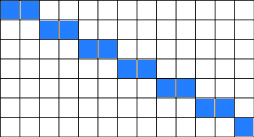If you need to only loop through the diagonal elements of the two-dimensional array you can use the following C# code (but should be more or less the same for any programming language):

``````int width = 5;
int height = 17;

bool[,] array = new bool[width, height];

var ratio = width / (float)height;

for (int i = 0, j = 0; i < width && j < height;)
{
array[i, j] = true; // only diagonal elements

if ((i+1) / (float) (j+1) <= ratio)
{
i++;
if (ratio <= 1)
j++;
}
else
{
j++;
if (ratio >= 1)
i++;
}
}``````

This is how it will look for "wide" array (width > height):and for "tall" array (height > width):

``````xoooo
xoooo
xoooo
xoooo
oxooo
oxooo
oxooo
ooxoo
ooxoo
ooxoo
ooxoo
oooxo
oooxo
oooxo
oooox
oooox
oooox``````

and for "square" array (width == height):

``````xooooo
oxoooo
ooxooo
oooxoo
ooooxo
ooooox``````